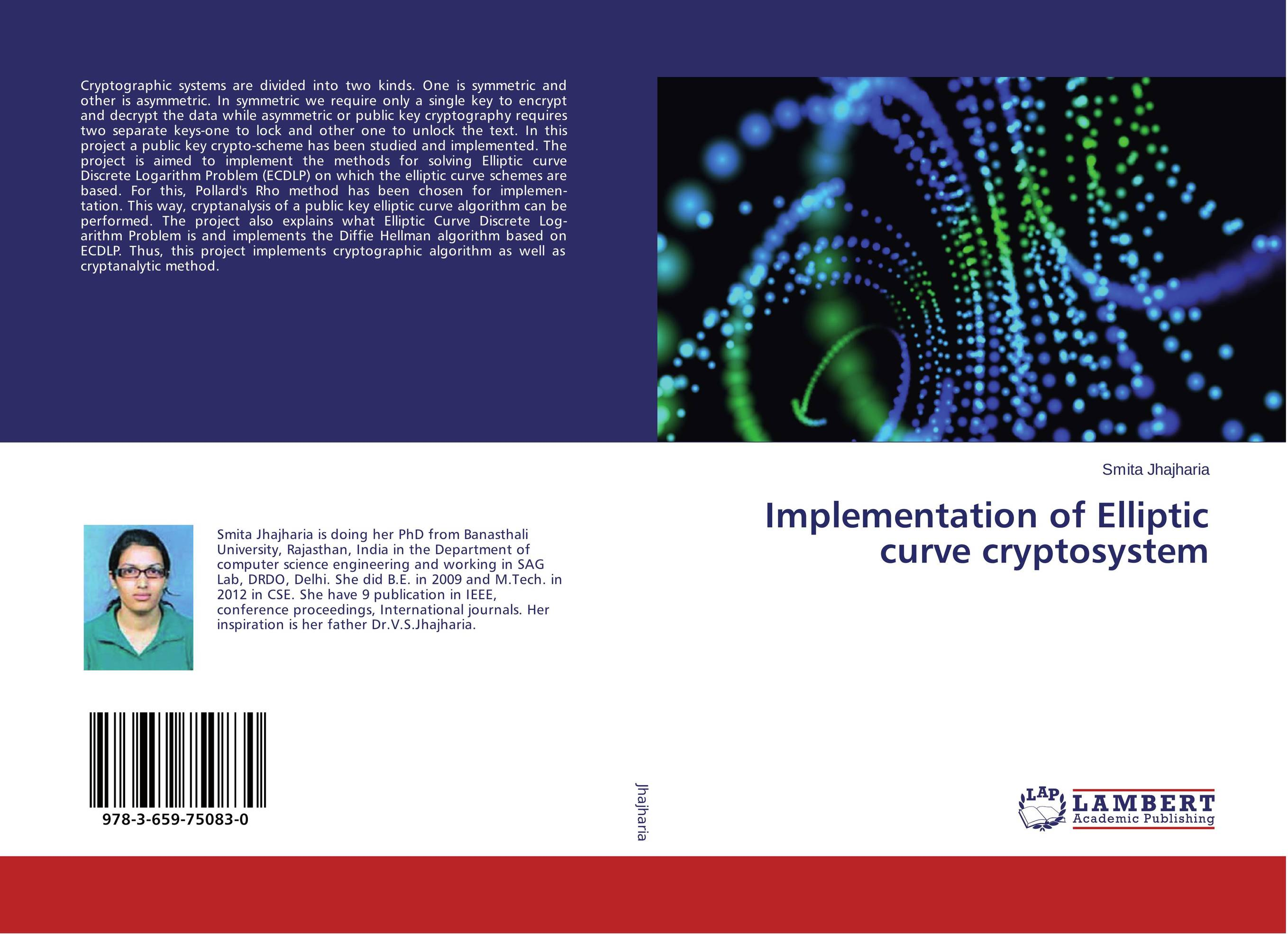# Implementation of Elliptic curve cryptosystem

###### Аннотация:
Cryptographic systems are divided into two kinds. One is symmetric and other is asymmetric. In symmetric we require only a single key to encrypt and decrypt the data while asymmetric or public key cryptography requires two separate keys-one to lock and other one to unlock the text. In this project a public key crypto-scheme has been studied and implemented. The project is aimed to implement the methods for solving Elliptic curve Discrete Logarithm Problem (ECDLP) on which the elliptic curve schemes are based. For this, Pollard's Rho method has been chosen for implemen- tation. This way, cryptanalysis of a public key elliptic curve algorithm can be performed. The project also explains what Elliptic Curve Discrete Log- arithm Problem is and implements the Diffie Hellman algorithm based on ECDLP. Thus, this project implements cryptographic algorithm as well as cryptanalytic method.
###### Издания произведения:9783659750830

### Рекомендуем

#### Комментарии

Пока нет ни одного комментария
Вы должны войти для того что бы оставлять комментарии